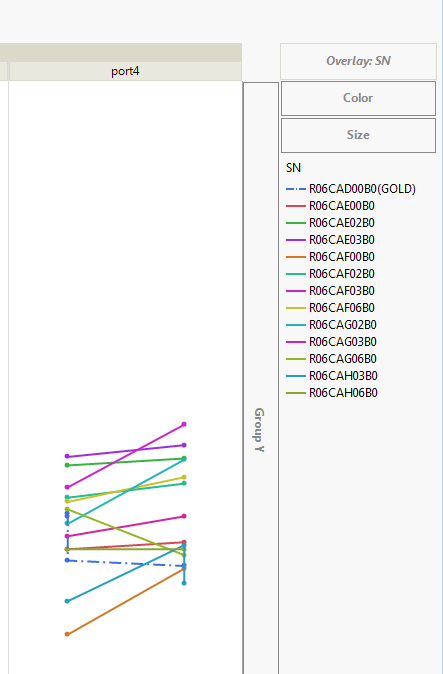Choose Language Hide Translation Bar
Highlighted

## How can I plot in graph builder like the attachment pictures?

I want plot the different line type with the condition which SN == “R06CAD00B0(GOLD)10 REPLIES 10
Highlighted

## Re: How can I plot in graph builder like the attachment pictures?

Go to the legend

Right click on the line you want to change

Select the line style you want

Jim
Highlighted

## Re: How can I plot in graph builder like the attachment pictures?

I knew this method,but I have many graph to change line type. how can I do it through script.

Highlighted

## Re: How can I plot in graph builder like the attachment pictures?

The easiest way to see how to do this, is to make the change to the graph, and then go to the red triangle and select "Save Script". The script it produces will give you the JSL required to make the change you need.
Jim
Highlighted

## Re: How can I plot in graph builder like the attachment pictures?

No，You didn't understand my what I meant， I want make one special SN with dotted line in many graph. Copy script can't do it.

Highlighted

## Re: How can I plot in graph builder like the attachment pictures?

I am sorry, I did not make my response very clear.  The instructions I gave you will provide you with the specific syntax required to make your suggested change.  I then assume that you would take that information, and build a script to your specifications that would use the syntax to make the changes to all of the graphs necessary.

Jim
Highlighted

## Re: How can I plot in graph builder like the attachment pictures?

txnelson，copy script only make specific position change its line type (example the first one number). I will change other SN, but this special SN will not be changed. Then the position of Special SN will changed. Do you clear what I said? I’m sorry for my poor English.
Highlighted

## Re: How can I plot in graph builder like the attachment pictures?

Here are two sample scripts that illustrate how you can use the Properties setting to change the line style for a specific value of SN.  The first one shows that position does not have to be specified to get the code to run correctly.  The second one shows that if you want to support the position, you can determine what the position should be, and then insert it into the code.

Names Default To Here( 1 );
dt = Open( "$SAMPLE_DATA\big class.jmp" ); // Change the age column to be character and change it's name to SN, // to mimic the SN in the graph from the first entry for the discussion dt:age << data type( character ) << set name( "SN" ); // Change the value of the age=15 to age=15(Gold) For Each Row( If( dt:SN == "14", dt:SN = "14(Gold)" ) ); // Create the code for the graph. Note, theGraph = Expr( Graph Builder( Variables( X( :sex ), Y( :height ), Overlay( :SN ) ), Elements( Line( X, Y, Legend( 9 ) ) ), SendToReport( Dispatch( {}, "400", ScaleBox, {Legend Model( 9, Properties( 0, {Line Style( "Dashed" )}, Item ID( "14(Gold)", 1 ) ) )} ) ) ); ); // Run the graph with all of the data theGraph; // Create a new table with no age 12 individuals, so the position of 15(Gold) // changes in the graph dt << select where( :SN != "12" ); dtNo12 = dt << subset( selected rows( 1 ), selected columns( 0 ) ); // Run the graph with the no 12 data theGraph; Names Default To Here( 1 ); dt = Open( "$SAMPLE_DATA\big class.jmp" );

// Change the age column to be character and change it's name to SN,
// to mimic the SN in the graph from the first entry for the discussion
dt:age << data type( character ) << set name( "SN" );

// Change the value of the age=15 to age=15(Gold)
For Each Row( If( dt:SN == "14", dt:SN = "14(Gold)" ) );

// Create the code for the graph.  Note,
theGraph = Expr(

// Find the position
Summarize( byGroup = by( :SN ) );
thePosition = Loc( byGroup, "14(Gold)" ) - 1;

Eval(
Substitute(
Expr(
Graph Builder(
Variables( X( :sex ), Y( :height ), Overlay( :SN ) ),
Elements( Line( X, Y, Legend( 9 ) ) ),
SendToReport(
Dispatch(
{},
"400",
ScaleBox,
{Legend Model(
9,
Properties( __pos__, {Line Style( "Dashed" )}, Item ID( "14(Gold)", 1 ) )
)}
)
)
)
),
Expr( __pos__ ), thePosition
)
);
);

// Run the graph with all of the data
theGraph;

// Create a new table with no age 12 individuals, so the position of 15(Gold)
// changes in the graph
dt << select where( :SN != "12" );

dtNo12 = dt << subset( selected rows( 1 ), selected columns( 0 ) );

// Run the graph with the no 12 data
theGraph;
Jim
Highlighted

## Re: How can I plot in graph builder like the attachment pictures?

txnelson, I plot graph with your first script,but the Dashed line is SN "12"，the script din't work. By the way, My JMP version is 11.

Highlighted

## Re: How can I plot in graph builder like the attachment pictures?

Did the second graph work?  I do not have access to JMP 11, so I can not test it.  There will be significant differences in Graph Builder from JMP 11 to JMP 14.

Jim
Article Labels

There are no labels assigned to this post.